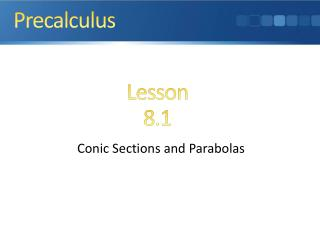DownloadDownload PresentationConic Sections and Parabolas

# Conic Sections and Parabolas

Télécharger la présentation## Conic Sections and Parabolas

- - - - - - - - - - - - - - - - - - - - - - - - - - - E N D - - - - - - - - - - - - - - - - - - - - - - - - - - -
##### Presentation Transcript

1. Precalculus Lesson 8.1 Conic Sections and Parabolas

2. Quick Review

3. Quick Review

4. What you’ll learn about • Conic Sections • Geometry of a Parabola • Translations of Parabolas • Reflective Property of a Parabola … and why Conic sections are the paths of nature: Any free-moving object in a gravitational field follows the path of a conic section.

5. Parabola A parabola is the set of all points in a plane equidistant from a particular line (the directrix) and a particular point (the focus) in the plane.

6. A Right Circular Cone (of two nappes)

7. Conic Sections and Degenerate Conic Sections

8. Second-Degree (Quadratic) Equations in Two Variables

9. Structure of a Parabola

10. Graphs of x2=4py

11. Parabolas with Vertex (0,0) • Standard equation x2 = 4pyy2 = 4px • Opens Upward or To the right or to the downward left • Focus (0,p) (p,0) • Directrixy = -px = -p • Axis y-axis x-axis • Focal length pp • Focal width |4p| |4p|

12. Graphs of y2 = 4px

13. Example Finding an Equation of a Parabola

14. Parabolas with Vertex (h,k) • Standard equation (x-h)2 = 4p(y-k)(y-k)2 = 4p(x-h) • Opens Upward or To the right or to the downward left • Focus (h,k+p) (h+p,k) • Directrixy = k-px = h-p • Axis x = h y = k • Focal length pp • Focal width |4p| |4p|

15. Example Finding an Equation of a Parabola

16. Homework: Text pg639/640 Exercises #2-20even